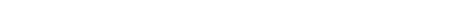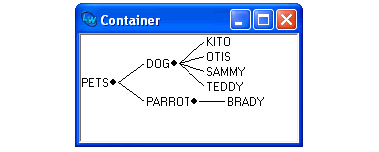### 6.7 Using graphs in your programs

You can read about the CAPI class `graph-pane` in the CAPI User Guide and Reference Manual for detailed API information for using graphs in your own programs. We will also look at a short example in this section. The following code listing defines a callback function and creates a graph-pane object:

`(defun node-children (node)`
`  (if (equal node 'pets)`
`      (list 'dog 'parrot)`
`    (if (equal node 'dog)`
`        (list 'Kito 'Otis 'Sammy 'Teddy)`
`      (if (equal node 'parrot)`
`          (list 'Brady)))))`
` `
`(setq test-graph`
`      (capi:contain`
`       (make-instance 'capi:graph-pane`
`                      :roots '(pets)`
`                      :children-function`
`                      'node-children)`
`       :best-width 300`
`       :best-height 400))`

The children function `node-children` should return `nil` for a leaf node in the graph or a list of child nodes for a non-leaf node. Sample Graph from a User Program shows the generated graph-pane.

Figure 6.12 Sample Graph from a User ProgramLispWorks IDE User Guide (Windows version) - 12 Feb 2015